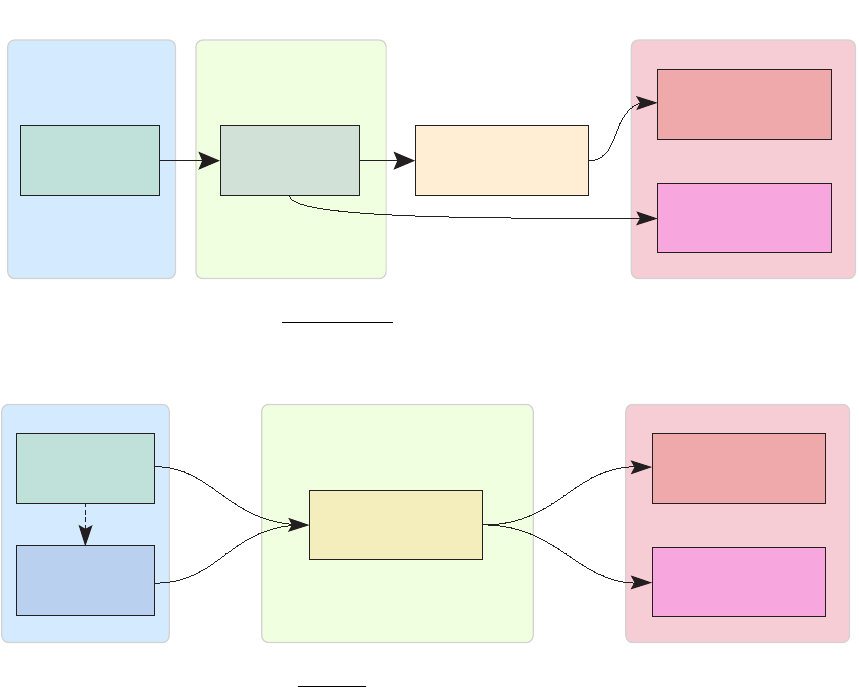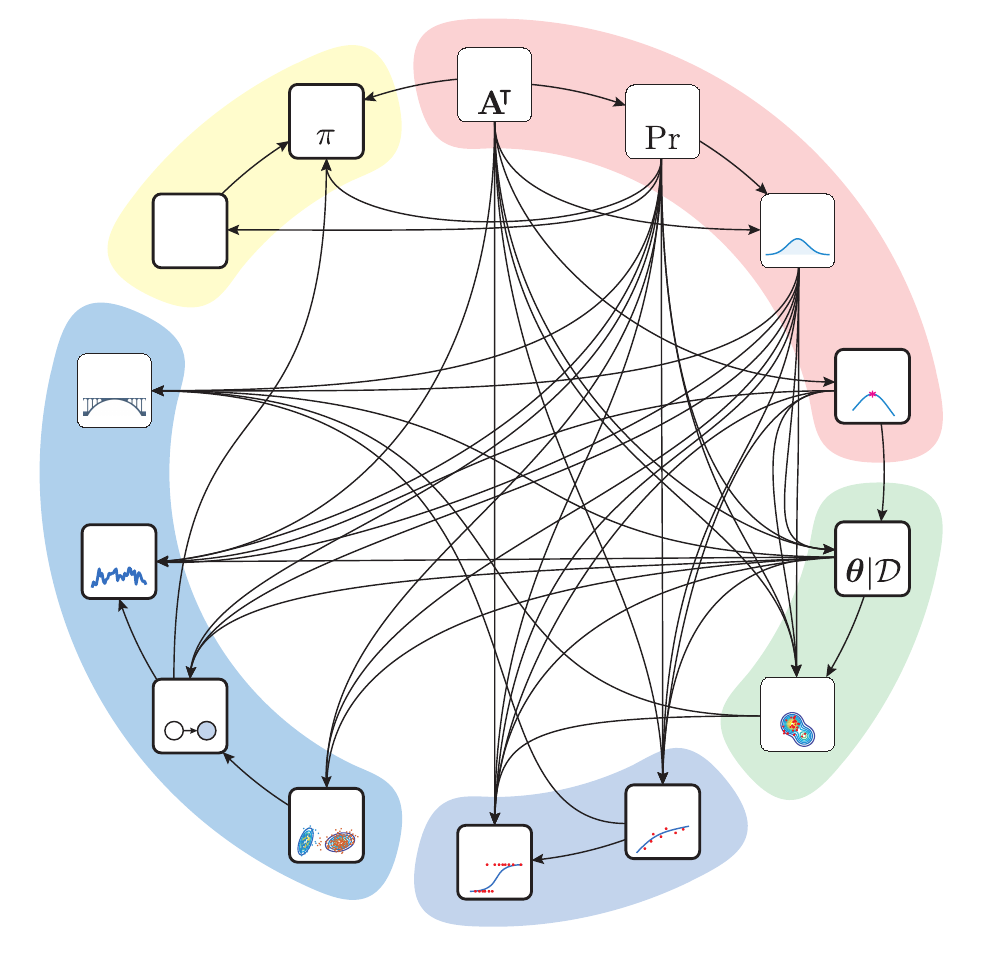1
Introduction
Machine learning (ML) describes a family of methods that allows
learning from data what relationships exist between quantities of
inte re st . The goal of learning relationships between quantities of
Civil engineering examples
Geotechnics:Fromasetofdiscrete
observations of soil resistances mea-
sured across space, we want to predict
the resistance for other unobserved
locations.
Environment:Foralake,wewant
to build a model linking the eect of
temperature and the usage of fertilizers
with the prevalence of cyanobacteria,
ﬁsh mortality, and water color.
Transportation :Wewanttopredict
the demand for public transportation
services from multiple heterogeneous
data sources such as surveys and transit
card usage.
on the displacement of a structure over
time, we want to detect the presence of
anomalies in its behavior.
inte re st is to gain information about how a system works and to
make predictions for unobserved quantitie s. This new knowledge
can t he n be employed to support decision making.
Learning from data is not new in the ﬁeld of engineering. As
depicted in ﬁgure 1.1a, without machine learning, the task of
learning is typically the role of the engineer, who has to ﬁgure out
the relationshi ps between the variables in a data set, t h en build
a hard-coded model of the syste m , and then return predictions
by evaluating this model with the help of a computer. The issue
with hard-coding rul es and equations is that it becomes increasingly
dicult as the number of factors considered increases. Figure
1.1b depicts how, with machine learning, the role of the human
is now shifted from learning to programming the computer so
Data Human
Computer
Predictions
Information
Inputs Learning Outputs
(a) Without machine learning
Data
Human
Computer
Predictions
Information
Inputs Learning Outputs
(b) With machine learning
Figure 1.1: Comparison of how, in the
context of engineering, relationships are
learned from data with and without using
machine learning.j.-a. goulet 2
it can learn. The question is, How do we program a computer
to learn? The quick answer is this: by deﬁning a generic model
that can be adapted to a wide array of problems by changing its
parameters and variables. For most machine learning methods,
learning consists in inferring these parameters and variables from
Note:
For a generic mo del
y
=
g
(
x
;
),
x
and
y
are the input and output variables
of interest that are evaluated by the model.
is a parameter characterizing the model
behavior.
data. Notwithstanding the speciﬁciti es for reinforcement learning
that we will cover later, the big picture is the following: learning
about parameters and variables is done by quantifying, for a given
set of their values, how good the model is at predicting observed
to make a choice: either pick a single set of values (that is, those
leading to the best predicti on s) or consider all possible values
that are compatible with data. In ﬁgure 1.1b, the dashed arrow
represents t h e practical reality where ML typically relies on a
human decision for the selection of a particular mathematical
method adapted to a given data set and problem.
One term often employed together with machine learning is ar -
tiﬁcial intelligence (AI). ML and AI are closely i nterconnected, yet
they are not synonyms. In its most general form, artiﬁcial intel-
ligence consists in the reproducti on of an intelligent behavior by
a machine. AI typically involves a system that can perceive and
inte rac t dynamically with its environment through the process
of making rational decisions. Note that such an AI system does
not have to take a physical form; in most c ases it is actually only
a computer program. In AI systems, decisions are typically not
hard-coded or learned by im it at i on ; instead, the AI system chooses
actions with the goal of maximizing an objective function that
is given to it. Behind such an AI system that interacts with its
envir on ment are machine learning methods that allow extracting
information from observations, predict future system responses,
and choose the optimal action to take. As depicted in the Venn dia-
gram in ﬁgure 1.2, machine learning is part of the ﬁeld of artiﬁcial
intell i gen ce .
ML
AI
Figure 1.2: The ﬁeld of artiﬁcial intelli-
gence (AI) includes the ﬁeld of machine
learning (ML).
Now, going back to machine l ear n in g, why opt for a probabilistic
approach to it? It is because the task of learning is intrinsically
uncertain. Uncer t ainties arise from the imperfect models employed
to learn from data, because data itself often involves imperfect
observations. Therefore, probabilities are at the core of machine
learning methods for representing the uncertainty associated with
our lack of knowledge. If we do not want to use machine learning as
a black box, but instead to under st an d how it works, going through
the probabilistic path is essential.
In machine learning, there are three main sub ﬁe l ds : supervised
probabilistic machine learning for civil engineers 3
learning, unsupervised learning, and reinforcement learning. Su-
pervised learning applies in the context where we want to build a
model describing the relationships between the characteristics of a
system deﬁned by covariates and observed system res ponses that
Note:
We employ the generic term system
to refer to either a single object or many
interconnected objects that we want to
study. We use the term covariates for
variables describing the characteristics or
the properties of a system.
are typically either continuous values or categories. With unsuper-
vised learning, t h e objective is to discover structures , patterns, sub-
groups, or even anomalies without knowing what the right answer is
because the target outputs are not observed. The third subﬁeld is
reinforcement learning, which involves more abstract concept s than
supervised and unsupervised learning. Reinforcement learning deals
with sequential decision problems where the goal is to learn the
optimal action to choose, given the knowledge that a system is in
a particular state. Take the example of infrastructure maintenance,
where, given the state of a structure today, we must choose between
performing maintenance or doing nothing. The key is that there is
no data to train on with respect to the d eci s ion -m aki n g behavior
that the computer should reproduce. With reinforcem ent learning,
the goal is to identify a policy describing the optimal act i on to
perform for each possible state of a system in order to maximize
the long-term accumulation of rewards. Note that the cl ass i ﬁc ati on
of machine learning methods within supervised, u n su pervised, and
reinforcement learning has limitations. For many met hods, the
frontie r s are blurre d because there is an overlap between more than
one ML subﬁeld with respect to the mathematical formulations
employed as wel l as the applications.
This book is intended to help making machine learning concepts
accessible to civil engineers who do not have a specialized back-
ground in statistics or in computer science. The goal is to dissect
and simplify, through a step-by-step review, a selection of key ma-
chine learning concepts and methods. At the end, the reader should
have acquired sucient knowledge to understand dedicated ma-
chine learning literatur e from which this book borrows and thus
expand on advanced methods that are beyond the scope of this
introductory work.
The diagram in ﬁgure 1.3 depicts the organization of this book,
where arrows represent the depen de nc i es between dierent chapters.
Colored regions indicate to which machine learning subﬁeld each
chapte r belongs. Before introducing the fundamentals associated
with each machine learning subﬁeld in Parts II–V, Part I covers
the background knowle dge required to understand machine learn-
ing. This background knowledge includes linear algebra (chapter
2), where we review how to harness the potential of matrices to
describe systems; probability theory (chapter 3) and probabilityj.-a. goulet 4
2
3
4
5
6
7
8
9
11
12
10
14
\$
15
13
B
a
c
k
g
r
o
u
n
d
B
a
y
e
s
i
a
n
E
s
t
i
m
a
t
i
o
n
S
u
p
e
r
v
i
s
e
d
L
e
a
r
n
i
n
g
U
n
s
u
p
e
r
v
i
s
e
d
L
e
a
r
n
i
n
g
R
e
i
n
f
o
r
c
e
m
e
n
t
L
e
a
r
n
i
n
g
Figure 1.3: Each square node describes a
chapter, and arrows represent the depen-
group chapters into the ﬁve parts of the
book. Note that at the beginning of each
part, this diagram is broken down into
subparts in order to better visualize the
dependencies between the current and
previous chapters.
distributions (chap t er 4) to describe our incomplete knowledge; and
convex optimization (chapter 5) as a ﬁrst method for allowing a
computer to learn about model parameters.
Part I I ﬁrst covers Bayesian estimation (chapter 6), which is
behind the formulation of supervised and unsupervised learning
problems. Second, it covers Markov chain Monte Carlo (MCMC)
methods (chapter 7), allowing one to perform Bayesian estimation
in c omp le x cases for which no analytical solution is available.
Part I II explores methods and concepts associated with super-
vised lear ni n g. Chapter 8 covers regression methods, where the goal
is to build models describing continuous-valued system responses as
a function of covariates. Chapter 9 presents classiﬁcation methods,
which are analogous to regression except that the system responses
are cat e gori e s rather than continuous values.
Part IV introduces the notions associated with unsupervised
learning, where the task is to build models that can extract the
underlying str uc t ur e present in data without having access to
direct observations of what this underlying structure should be.
In chapter 10, we ﬁrst approach u ns upervised learning through
clustering and dimension reduction. For clustering, the task is to
identify sub gr oup s within a set of observed covariates for which
probabilistic machine learning for civil engineers 5
we do not have access to the subgroup labels. The role of dimen-
sion reduction is, as its name implies, to reduce the number of
dimensions requi r ed to represent data while minimizing the loss
of information. Chapter 11 presents Bayesian networks,which
are graph-based probabilistic methods for modeling dependencies
within and between systems through their joint probability. Chapter
12 presents state-space models, which allow creating probabilistic
models for time-dependent systems using sequences of observations.
Finally, chapter 13 presents how we can employ the concepts of
probabilistic i n fe re nc e for the purpose of model calibration.Model
calibration refe rs to the task of using observations t o improve our
knowle dge associated with hard-coded mathematical models that
are commonly employed in engineering to describe systems. This
application is classiﬁed unde r the umbrella of unsupervised learning
because, as we will see, the main task consists in inferring hidden-
state variables and parameters, for which observations are not
available.
Part V presents the fundamental notions necessary to deﬁne
reinforcement learning problems. First, chapter 14 presents how
rational decision s are made in uncertain contexts using the utility
theory. Chapter 15 presents how to e x te nd rational deci si on making
to a sequential context using the Markov decision process (MDP).
Finally, building on the MDP theory, we introduce the fundamental
concepts of reinforcement learning, where a virtual agent learns how
to take optimal decisions through trial and e r ror while interacting
with its environment.